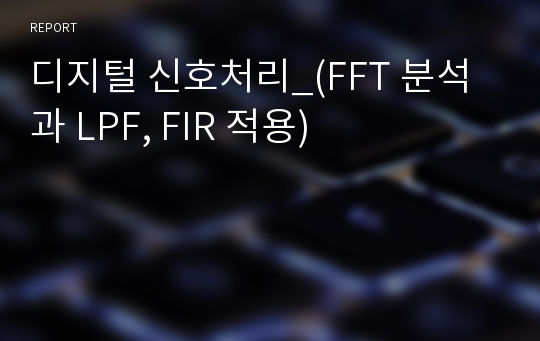# 디지털 신호처리_(FFT 분석과 LPF, FIR 적용)

최초 등록일
2016.08.22
최종 저작일
2014.06
17페이지/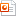MS 파워포인트
가격
판매자kei**** 2회 판매
다운로드
장바구니

## 목차

1. 디지털신호의 FFT (Fast Fourier Transform) 분석
2. 디지털신호에 LPF (Low Pass Filter) 적용
3. 디지털신호에 FIR (Finite Impulse Response) 적용

## 본문내용

Feature of Fitter

FFT ( Fast Fourier transform)
Fourier analysis converts time to frequency and vice versa.
An FFT rapidly computes such transformations by factorizing the DFT matrix into a product of sparse factors.
As a result , fast fourier transforms are widely used for many applications in engineering, science, and mathematics.

LPF ( Low Pass Filter)
A low-pass filter is a filter that passes low-frequency signals and attenuates (reduces the amplitude of) signals with frequencies higher than the cutoff frequency.

없음

## 자료문의

ㆍ이 자료에 대해 궁금한 점을 판매자에게 직접 문의 하실 수 있습니다.
ㆍ상업성 광고글, 욕설, 비방글, 내용 없는 글 등은 운영 방침에 따라 예고 없이 삭제될 수 있습니다.
ㆍ다운로드가 되지 않는 등 서비스 불편사항은 고객센터 1:1 문의하기를 이용해주세요.

## 판매자 정보kei****
회원 소개글이 없습니다.
판매지수
ㆍ판매 자료수
39
ㆍ전체 판매량
30
ㆍ최근 3개월 판매량
1
ㆍ자료후기 점수
평균
ㆍ자료문의 응답률
받은문의없음
판매자 정보
ㆍ학교정보
• 비공개
ㆍ직장정보
• 비공개
ㆍ자격증
• 비공개
• 위 정보 및 게시물 내용의 진실성에 대하여 해피캠퍼스는 보증하지 아니하며, 해당 정보 및 게시물 저작권과 기타 법적 책임은 자료 등록자에게 있습니다.
위 정보 및 게시물 내용의 불법적 이용, 무단 전재·배포는 금지되어 있습니다.
저작권침해, 명예훼손 등 분쟁요소 발견시 고객센터의 저작권침해 신고센터를 이용해 주시기 바랍니다.

## 찾던 자료가 아닌가요?아래 자료들 중 찾던 자료가 있는지 확인해보세요

•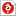디지털신호처리 (DSP) FIR Filter Design 학 과 : 과 ... ]; F_x=fft(x_n); plot(f,abs(F_x)); 5. Plot ... W=[180 220]/fs*2; M=80; b=fir1(M,W
•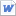를 취득함에 있어서 노이즈가 끼었다고 가정하여 디지털 신호처리를 한 신호 ... 같다. 그림 3 식 (1) 위의 신호디지털 신호처리를 수행하기 위해 A ... /D converter를 통해 디지털 신호로 바꾸어야 한다. A/D
•05 디지털 신호처리 고찰사항 06 목적 01 Filter 가 무엇인지 ... 알고 , 디지털 필터를 설계해 본다 . 디지털 신호처리 Filter 란 ... 처리 디지털 필터 는 특정한 성질의 결과를 얻기 위해서 이산신호처리
•디지털 신호처리와 디지털 필터 3페이지
디지털 신호처리디지털 필터 DSP(Digital Signal ... 시스템이 보편적으로 보급 및 이용됨에 따라 디지털 신호 처리의 필요성과 ... 중요성은 증가하고 있다. 디지털 신호 처리디지털화된 신호를 사용자가
•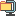" using 'FFT'. Plot the spectrum ... "stem". >> stem(abs(FFT(KTXSignal))) 2 ... , abs(FFT(KTXSignal))) 3. Plot the
•이용하여 디지털 신호 처리의 시스템 형태 중 디지털 필터, 그 중에서도 ... : Using MATLAB 매트랩을 이용한 신호처리 MATLAB은 신호 처리 ... FIR Filter - Window Function Method
•디지털신호처리과목 DSP필터설계 12페이지
/(Fs/2); B = fir1(M,wc); [H, w] = freqz(B,1 ... ,1,x); L = Fs/fm*10; Xk = fftshift(fft(x ... , L)); Yk = fftshift(fft(y, L)); figure
더보기최근 본 자료더보기